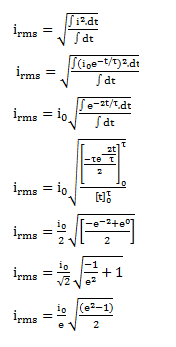# The current in the discharging LR circuit is given by

Question:

The current in the discharging LR circuit is given by $\mathrm{i}=\mathrm{i}_{0} \mathrm{e}^{-\mathrm{t} / \tau}$ where $T$ is the time constant of the circuit. Calculate the rms current for the period $t=0$ to $t=T$.

Solution: